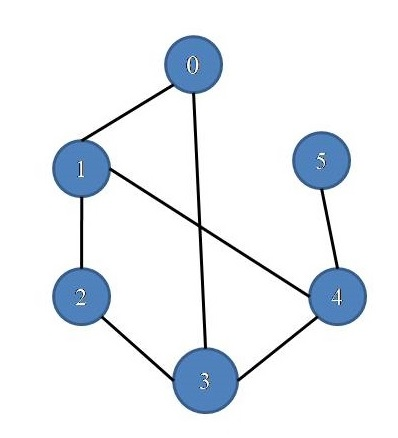# Count all the possible path between two vertices

In this article, we are going to see how to find number of all possible paths between two vertices?
Submitted by Souvik Saha, on March 26, 2019

What to Learn?

How to count all possible paths between two vertices?

In the graph there are many alternative paths from vertex 0 to vertex 4

```    1.	0 → 1 → 2 → 3 → 4
2.	0 → 1 → 4
3.	0 → 3 → 2 → 1 → 4
4.	0 → 3 → 4
```Algorithm:

Here we use a recursive method to detect all the paths of a graph,

1. We start the recursive function with the starting vertex.
2. For each node
1. Whenever we visited one vertex we mark it and go for all its unvisited adjacent nodes.
2. If we found the destination vertex then count the number else we go for recursive call.
```    Check(current node){
visit[curr_node]=true;
if(curr_node == destination){
count++;
}
else{
for( all the adjacent vertices ){
if(not visited yet) then
}
}
}
```

C++ implementation:

```#include <bits/stdc++.h>
using namespace std;

//Make a pair between vertex x and vertex y
ls[x].push_back(y);
ls[y].push_back(x);
}

//count the number of paths exists
void path_count(list<int>*ls,int s,int d,bool *visit,int &count){
visit[s]=true;
if(s==d){
count++;
}
else{
list<int>::iterator it;
for(it=ls[s].begin();it!=ls[s].end();it++){
if(!visit[*it]){
path_count(ls,*it,d,visit,count);
}
}
}
visit[s]=false;
}

int main()
{
list<int> ls;

bool visit;

for(int i=0;i<6;i++){
visit[i]=false;
}

int count=0;
path_count(ls,0,4,visit,count);
cout<<"Paths are : "<<count<<endl;

return 0;
}
```

Output

```Paths are : 4
```

Top MCQs

Languages: » C » C++ » C++ STL » Java » Data Structure » C#.Net » Android » Kotlin » SQL
Web Technologies: » PHP » Python » JavaScript » CSS » Ajax » Node.js » Web programming/HTML
Solved programs: » C » C++ » DS » Java » C#
Aptitude que. & ans.: » C » C++ » Java » DBMS
Interview que. & ans.: » C » Embedded C » Java » SEO » HR
CS Subjects: » CS Basics » O.S. » Networks » DBMS » Embedded Systems » Cloud Computing
» Machine learning » CS Organizations » Linux » DOS
More: » Articles » Puzzles » News/Updates